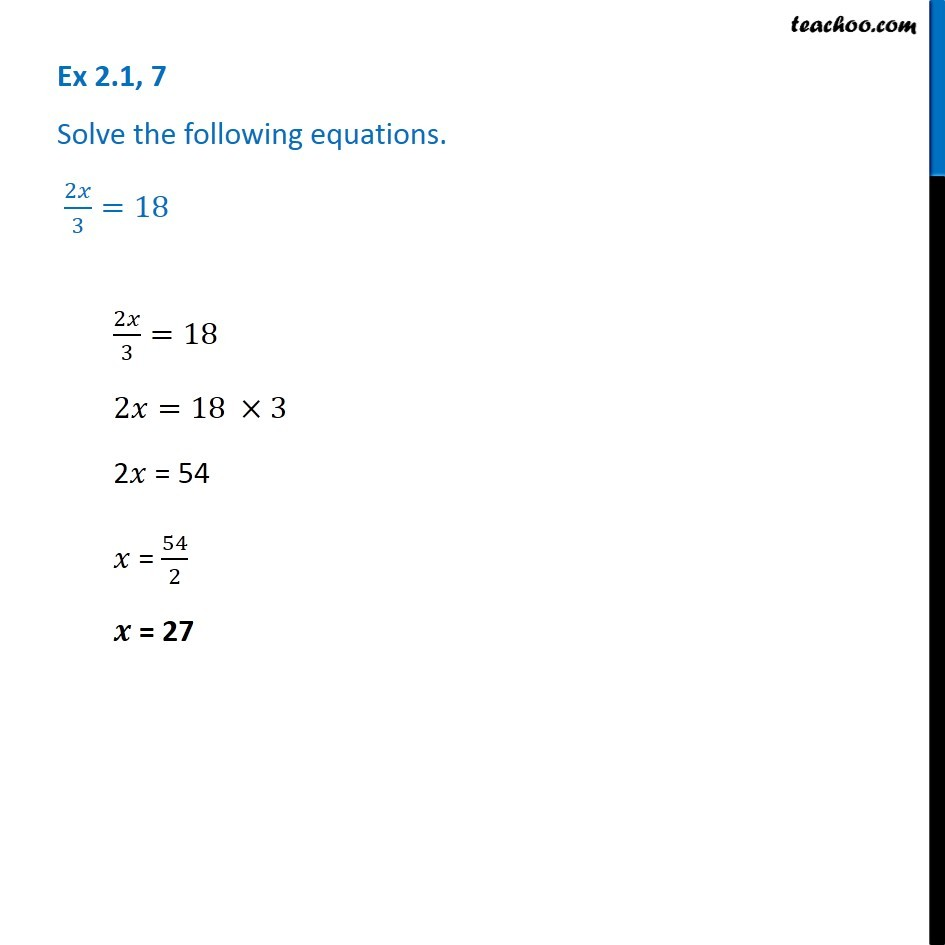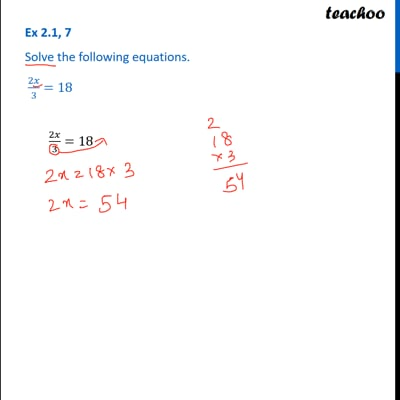Ex 2.1

Chapter 2 Class 8 Linear Equations in One Variable
Serial order wiseThis video is only available for Teachoo black users

Get live Maths 1-on-1 Classs - Class 6 to 12

### Transcript

Ex 2.1, 7 - Chapter 2 Class 8 - Linear Equations in one variable - NCERT Book Solve the following equations. 2x/3 = 18 2x/3 = 18 2x = 18 ×3 2x = 54 x = 54/2 x = 27